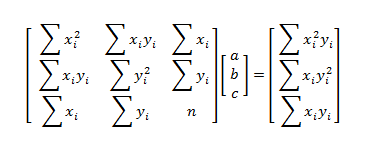# Rational Function Regression Calculator: y = (ax + c)/(x - b)

Enter X and Y Data Pairs Below
 X Y
 X Y
 X Y

#### Rational Function Regression y = (ax + c)/(x - b)

One of the simplest rational functions is the ratio of two linear functions. Rational functions of the form y = (ax + c)/(x - b) are a good choice to model data that levels off after a period of time without oscillations. The horizontal asymptote of this function is y = a, the vertical asymptote is x = b, and the y-intercept is -c/b.

In the form y = (ax + c)/(x - b), the function is not linear in the parameters a, b, and c. However, the equation can be transformed with simple algebra:

y = (ax + c)/(x - b)
(x - b)y = ax + c
xy - by = ax + c
xy = ax + by + c

This equation is linear in the variables a, b, and, c, so you can use the least squares method to find the "best fit" values of a, b, and c. Best fit in the least squares sense means minimizing the equation

F(a, b, c) = ∑(xiyi - axi - byi - c)²,

which amounts to solving the system ∂F/∂a = 0, ∂F/∂b = 0, and ∂F/∂c = 0. The solution can be found with matrix math.

#### Determining a, b, and c with Matrices

The matrix equation for simple rational regression iswhere n is the number of data pairs (xi, yi). So long as the 3-by-3 matrix on the left is invertible, there is a unique solution (a, b, c) that minimizes the function F(a, b, c) and provides the parameters for the best fit rational function.

#### Example

Find the equation of a rational function that fits the points

(0, 20) (0, 18.5) (0, 18)
(1, 10) (1, 10) (1, 9)
(2, 7) (2, 6.5) (2, 6)
(3, 5) (3, 4.5) (3, 4.5)
(4, 5) (4, 5) (4, 4.5)

Using the rational function regression calculator above, you can find that the equation

y = (2.2556x + 11.7798)/(x + 0.5922)

provides a reasonably good fit to the data.